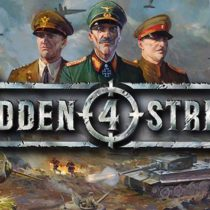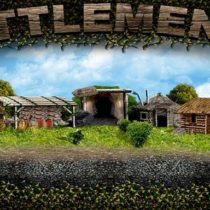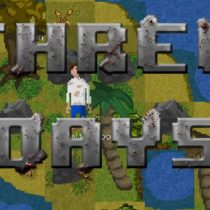#### Strategy GAMES• Strategy
• Simulation
• Casual
• Survival
• Sci-fi• Simulation
• Strategy• Strategy
• RTS• Strategy
• Anime
• RPG
• Simulation
• Visual Novel
• Turn-Based• Action
• Shooter
• Survival
• Open World
• Casual
• Strategy• Simulation
• Casual
• Strategy
• Management
• Building• Strategy
• Simulation
• RPG
• Management• Strategy
• Turn-Based• Simulation
• Strategy
• Action• RPG
• Strategy
• Anime• Casual
• Strategy
• Puzzle• Simulation
• Open World
• Strategy• Tower Defense
• Action
• Strategy
• Open World
• Survival
• RPG
• Sci-fi• Action
• Casual
• Simulation
• Strategy
• VR
• Shooter• RPG
• Strategy
• Open World• Casual
• RPG
• Simulation
• Strategy
• Survival• Simulation
• RPG
• Text-Based
• Strategy
• Horror